## Answer the following questions for a mass that is hanging on a spring and oscillating up and down with simple harmonic motion. Note: the osc

Question

Answer the following questions for a mass that is hanging on a spring and oscillating up and down with simple harmonic motion. Note: the oscillation is small enough that the spring stays stretched beyond its rest length the entire time. (top, equillibrium, bottom, top & bottom, or nowhere)

1. Where in the motion is the acceleration zero?
2. Where in the motion is the magnitude of the acceleration a maximum?
3. Where in the motion is the magnitude of the net force on the mass a maximum?
4. Where in the motion is the magnitude of the force from the spring on the mass zero?
5. Where in the motion is the magnitude of the force from the spring on the mass a maximum?
6. Where in the motion is the speed zero?
7. Where in the motion is the magnitude of the net force on the mass zero?
8. Where in the motion is the speed a maximum?

Yes or no for the next two.

1. When the object is at half its amplitude from equilibrium, is its speed half its maximum speed?
2. When the object is at half its amplitude from equilibrium, is the magnitude of its acceleration at half its maximum value?

in progress 0
2 weeks 2021-09-05T16:31:19+00:00 1 Answers 0 views 0

1. equilibrium

2. bottom

3. bottom

4. nowhere

5. bottom

6. top & bottom

7. equilibrium

8. equilibrium

1. No

2. Yes

Explanation:

According to the following equation of motion for SHM: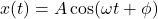where A is the amplitude, ω is the angular frequency, and ∅ is the phase angle.

Furthermore, the velocity and acceleration functions are as follows: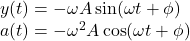1. The acceleration is zero at the equilibrium. At the equilibrium, the net force on the object is zero. And according to Newton’s Second Law, if the net force is zero, then the acceleration is zero as well.

2. The forces on the object in a vertical spring are the weight of the object and the spring force.

F = mg – kx

Since mg is constant along the motion, then the net force is maximum at the amplitude. For the special case in this question, the mass is always below the rest length of the spring. So the net force is maximum at the lower amplitude, because x is greater in magnitude at the lower amplitude.  According to Newton’s Second Law, acceleration is proportional to the net force, hence the acceleration is at a maximum at the bottom.

3. As explained above, the magnitude of the net force is at a maximum at the lower amplitude, that is bottom.

4. The spring force is defined by Hooke’s Law: F = -kx. Since the oscillation is small enough so that the mass is always below the rest length of the spring, then x is always greater than zero, hence nowhere in the motion will the spring force becomes zero.

5. As explained above, the force of gravity is constant and the spring force is proportional to the displacement, x. Therefore, the spring force is at a maximum at the lower amplitude, that is bottom.

6. The speed is zero when the mass is instantaneously at rest, that is the amplitude.

7. The net force on the mass is zero at the equilibrium.

8. The speed is at a maximum at the equilibrium.

1.  We will use the equation of motions given above. For simplicity, let’s take ∅ = 0. At half its amplitude: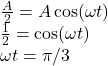Then the velocity at that point is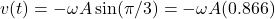The maximum speed is where the acceleration is equal to zero: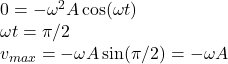Comparing the maximum velocity to the velocity at A/2 yields that it is not half the maximum velocity: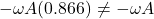2. The maximum acceleration is at the amplitude.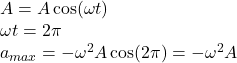And the acceleration at A/2 is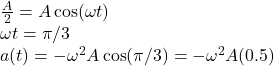Comparing these two results yields that the acceleration at half the amplitude is half the maximum acceleration.